## Why Is Excel Showing the Formula Instead of the Result?

There could be a few reasons why Excel is showing the formula instead of the result. First of all, all formulas in Excel must start with the equal sign “=”. Make sure there is nothing before the equal sign. Especially if there is an apostrophe ( ‘ ) in front, in Excel (and also in other programming languages), apostrophe turns a formula or a line of code into a comment or text so the program will not run the formula or code.

## Cell is in TEXT format

This is the most common reason why Excel is showing formulas instead of the results. It happens most often when a report is exported from another system, perhaps in a CSV format. When entering a formula, it will by default be in a TEXT format: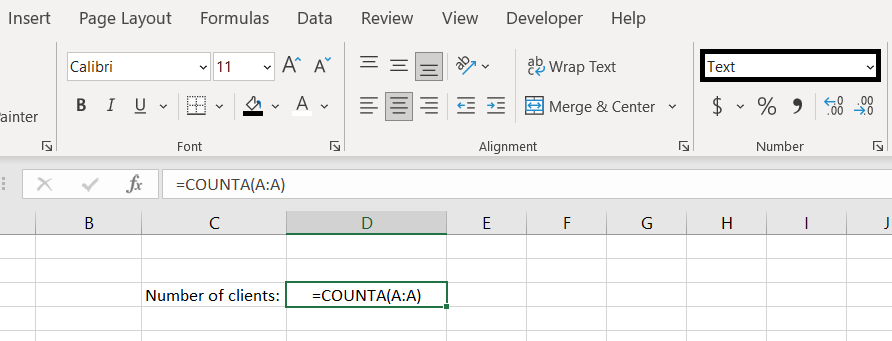To resolve this, change the format to General and enter the formula again: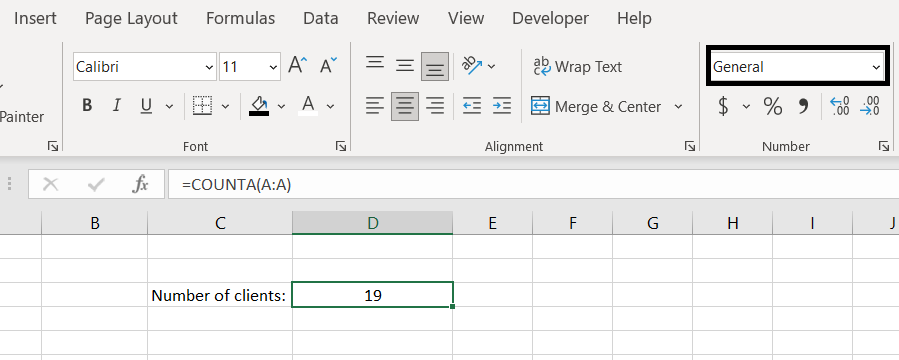## Show Formulas is Enabled

This is a less common explanation (but maybe someone is playing a prank on us, who knows?) If we go to the Formulas tab, we need to make sure Show Formulas is not selected: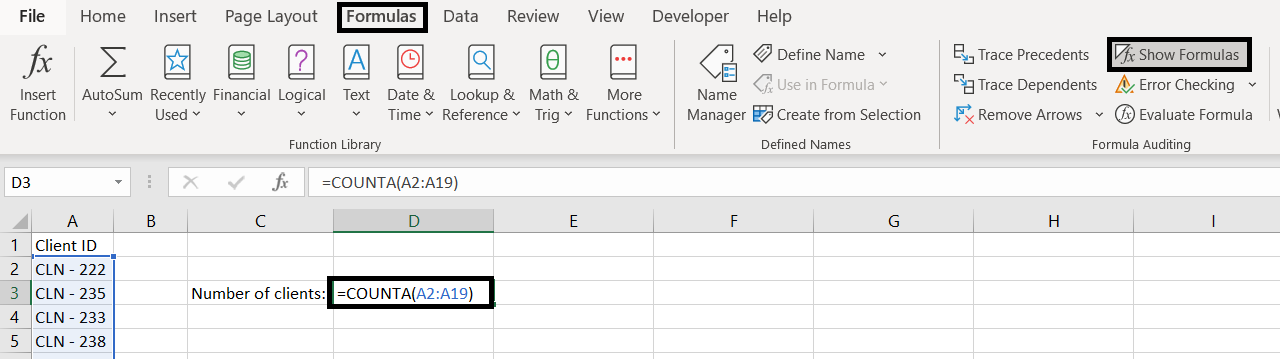Simply deselect the Show Formulas button and we shall see the end result of the formula instead of the formula itself.

## The Apostrophe – All Formulas Must Start With An Equal Sign

Excel will only recognize a function or a formula and calculate it if it starts with an equal sign. That means if in a cell, we type in “1+2”, Excel will not do the calculation for us. It will simply remain as “1+2”. To have Excel do a calculation, it must start with an equal sign. Using the same example, we must enter “=1+2”.

But what if we want to enter a formula or a function into a cell and do not want Excel to calculate it? Well here we can add an apostrophe in the beginning. And the apostrophe won’t appear in the cell. What the apostrophe does is that it tells Excel this is a text and not a formula to be calculated: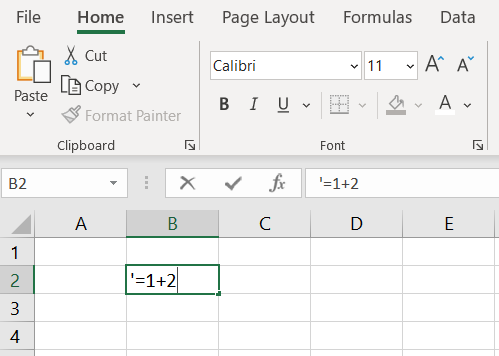Have you ever entered a value in Excel that starts with 0? For example: “007” and then Excel just removes the 0s for us and leaves the cell with 7. That is because Excel automatically recognizes “007” as a number. There are two ways we can change this and keep the 0s in front:

1. Change format of the cell into Text: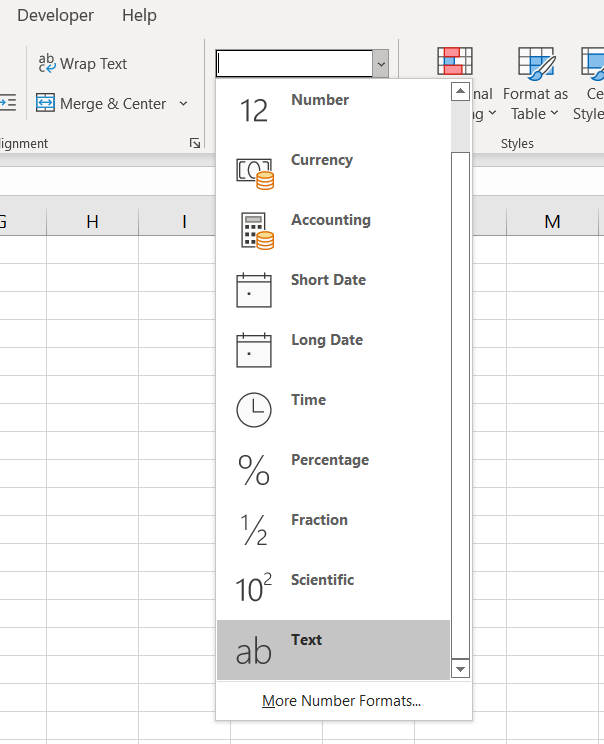With that, whatever we enter into the text, it will appear exactly the way we put it
2. Another way, a quicker way, would be to simply put an apostrophe before typing in the value. For example: ‘007. This will also have Excel recognize that this cell is now in a text format.

We hope this has helped you in understanding why formulas are not calculating and why Excel is showing the formulas and not calculating it. If you have gone through this article and none of these situations are applicable, feel free to leave us a comment below! We want to keep improving our content and make it more complete. So if there are other common scenarios where Excel is showing the formula instead of calculating it, please share with us!